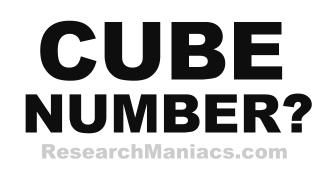Is 8 a cube number?

Cube Question: Is 8 a cube number?

For 8 to be a cube number the following equation must be true:

n3 = 8 where n is a whole number.

In other words, if n*n*n = 8, then 8 is a cube number.

If you set n=2, you get 2*2*2 which equals 8. Therefore, we can conclude that 8 is a cube number.

Answer: Is 8 a cube number? = YES

 Cube Number? Check other numbers to see if they are cube. List of Cube Numbers Here is a list of cube numbers.